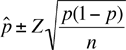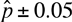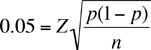I have to do a research about the behavior of library users. We are going to apply a survey to a population of 1280 students (Management an Economics students) but of course we have to do it to a sample....I need to know the size of my sample. Well, the information I know is that d= precision...and in this case d= 0.05 q= 1-p p= is the "expected ratio" (proportion esperada, in spanish)....I am not sure if this is 0.03 or 0.05 Z= is the Confidence Intervals. We use the 95% so Z= 1,96 I tried to use the formula...but I can't get a result. I haven't found a book with this formula so that I could see some examples. I don't know what other kind of information you need. I will be thankful if you can help me Ivonne Hi Ivonne, The confidence interval expression for the proportion in a binomial distribution isYou want the precision to be 0.05 and hence you want your confidence interval to beHenceIt is this expression that gives you the sample size. You know Z and you have an estimate for p (0.03 or 0.05) and hence you can find n. Squaring both sides and solving for n gives n = ( Z/0.05)2 p(1 - p) You are asked for a 95% confidence interval so Z = 1.96 and hence if the expected ratio is 0.03 then n = ( 1.96/0.05)2 0.03(1 - 0.03) = 44.7 With sample size estimates you must round up so I get a sample size of 45. Penny